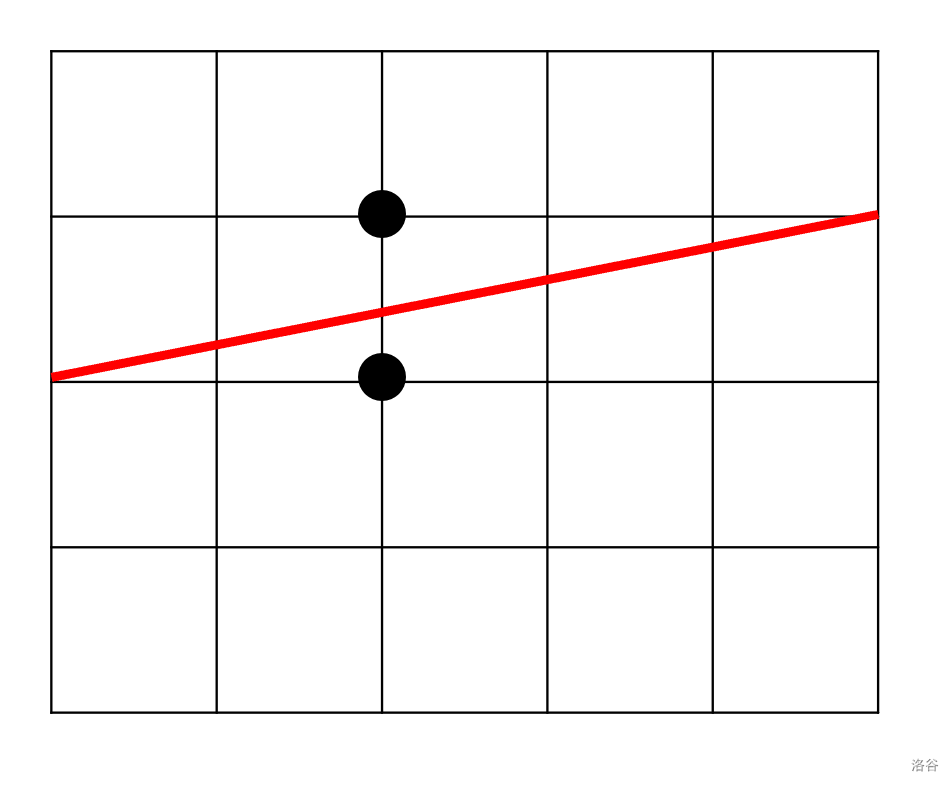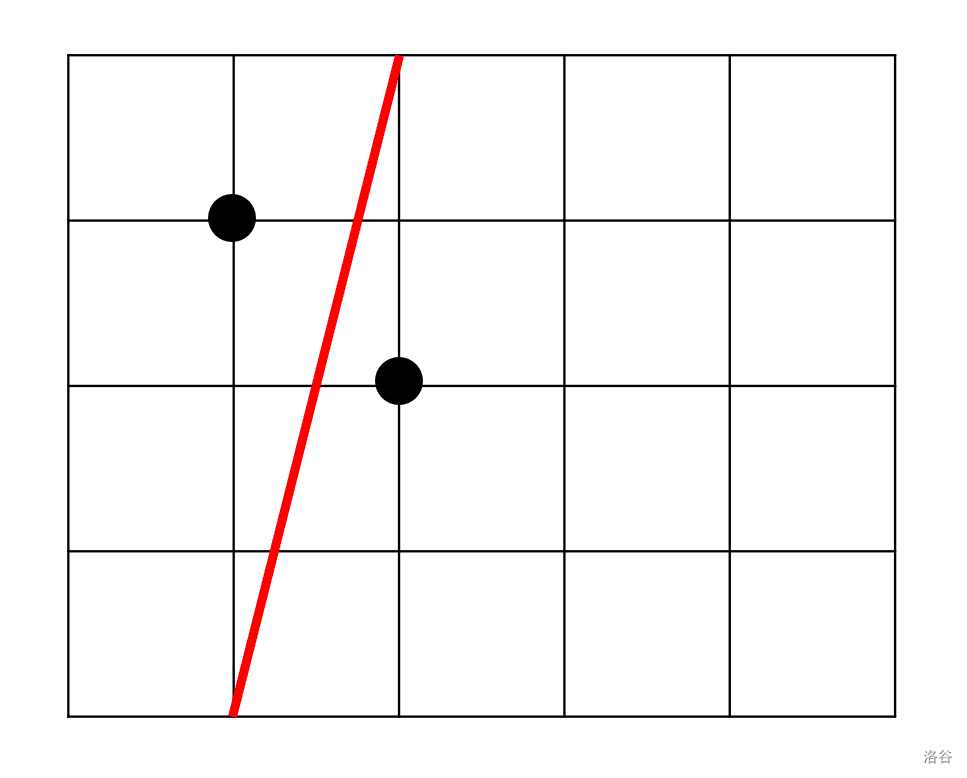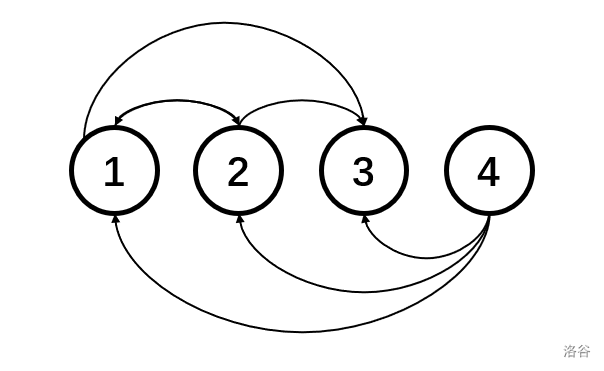2021.2.3 训练赛

A.Anniversary Cake

w,h \leq 10^9Code:

signed main() {
//FO(anniversary)
if (x1 == x2) cout << 0 << " " << y1 << " " << w << " " << y2;
else cout << x1 << " " << 0 << " " << x2 << " " << h;
return 0;
}

C.CodeCoder vs TopForces

n 个人，每个人有两个能力值 a_ib_i。一个人能打败另一个人当且仅当这个人有一项或两项能力值都大于另一个人。也就是说当 A 比 B 强时可能有 B 比 A 强。

n \leq 10^5,a_i,b_i \leq 10^6O(n^2) 做法，对于每个点 dfs 一遍，得出每个点的可达点数量。

Code:


struct node {
int val,pos;
}a[N],b[N];

int n;

bool cmp(node p,node q) {
return p.val < q.val;
}

vector <int> G[N];

int vis[N],ans,orz[N];

void dfs(int x) {
if (!vis[x]) ++ans;
vis[x] = 1;
for (int i = 0;i < G[x].size();++i) {
int y = G[x][i];
if (!vis[y]) dfs(y);
}
}

signed main() {
for (int i = 1;i <= n;++i) {
a[i].pos = i,b[i].pos = i;
}
sort(a+1,a+1+n,cmp),sort(b+1,b+1+n,cmp);
for (int i = 2;i <= n;++i) {
G[a[i].pos].push_back(a[i-1].pos),G[b[i].pos].push_back(b[i-1].pos);
}
for (int i = 1;i <= n;++i) {
if (!vis[a[i].pos]) dfs(a[i].pos);
orz[a[i].pos] = ans - 1;
}
for (int i = 1;i <= n;++i) {
printf("%d\n",orz[i]);
}
return 0;
}

F.Folding

1\le W,H,w,h\le10^9

Code:

int solve(int y,int x) {
if (x == y) return 0;
int cnt = 0;
while (1) {
x *= 2;++cnt;
if (x >= y) return cnt;
}
}

signed main() {
//FO(folding)
int ans = 0;
l1 = min(a,b),h1 = max(a,b),l2 = min(c,d),h2 = max(c,d);
if (l2 > l1 || h2 > h1) {
printf("-1");return 0;
}
ans = min(solve(l1,l2) + solve(h1,h2),solve(l1,h2) + solve(h1,l2));
printf("%d",ans);
return 0;
}

G.Gangsters in Central City 给出一棵含有 n 个节点的，且 1 为根节点的树，叶子节点都是房屋，让你在一个集合里面进行添加房屋和移除房屋的操作。

1. 最少需要砍多少条边，才能使已选房屋都不能从根节点到达。

2. 在第 1 问的条件下，如何砍边使从根节点点开始走不能到达的非已选房屋数目最小，输出最小值。

n \leq 10^5

1. 哪些点算是集合中靠的比较近的点？
2. 怎么找到多个点的 LCA？

void dfs2(int x,int anc) {
bel[x] = anc;//bel 记录的是这个点属于哪个
for (int i = 0;i < G[x].size();++i) {
int y = G[x][i];
dfs2(y,anc);
}
}
//in main
for (int i = 1;i < n;++i) {
int x;cin >> x;G[x].push_back(i+1);
deg[x]++;
if (x == 1) lis.push_back(i+1);//找出所有和根节点直接相连的点丢进vector里面
}
for (int i = 0;i < lis.size();++i) {
dfs2(lis[i],lis[i]);
}

Code:

int bel[N];//这个点属于哪个子树
int rul[N],dfn[N],cnt,deg[N],vis[N],rk[N];//这个点管辖了几个叶子
int n,q;
bool leaf[N];

vector <int> G[N],lis;

set <int> s[N];

void dfs1(int x) {
dfn[x] = ++cnt;rk[cnt] = x;//求出每个点dfs序以及每个dfs序对应的点
for (int i = 0;i < G[x].size();++i) {
int y = G[x][i];
dfs1(y);
}
}

int dfs2(int x,int anc) {
//printf("%d %d\n",x,anc);
bel[x] = anc;
if (leaf[x]) {//求出每个点控制了多少个叶子节点
return rul[x] = 1;
}
for (int i = 0;i < G[x].size();++i) {
int y = G[x][i];
rul[x] += dfs2(y,anc);
}
return rul[x];
}
//树剖求LCA
int dep[N],top[N],siz[N],fat[N],son[N],ans1,ans2;

void dfs3(int x,int f) {
siz[x] = 1,fat[x] = f,dep[x] = dep[f] + 1,son[x] = 0;
for (int i = 0;i < G[x].size();++i) {
int y = G[x][i];
dfs3(y,x);
siz[x] += siz[y];
if (siz[y] > siz[son[x]]) {
son[x] = y;
}
}
}

void dfs4(int x,int f) {
if (son[f] == x) top[x] = top[f];
else top[x] = x;
if (son[x]) dfs4(son[x],x);
for (int i = 0;i < G[x].size();++i) {
if (G[x][i] != son[x]) dfs4(G[x][i],x);
}
}

int LCA(int x,int y) {
while (top[x] != top[y]) {
if (dep[top[x]] < dep[top[y]]) swap(x,y);
x = fat[top[x]];
}
return (dep[x] < dep[y]) ? x : y;
}

signed main() {
cin >> n >> q;
for (int i = 1;i < n;++i) {
int x;cin >> x;G[x].push_back(i+1);
deg[x]++;
if (x == 1) lis.push_back(i+1);//先将直接相连的都丢到一个vector里
}
for (int i = 1;i <= n;++i) {
if (!deg[i]) leaf[i] = 1;//维护所有叶子节点
}
for (int i = 0;i < lis.size();++i) {
dfs2(lis[i],lis[i]);
}
dfs1(1),dfs3(1,0),dfs4(1,0);
int now = 0,las;
while (q--) {
char opt;int x;
cin >> opt >> x;
//now保存的是集合里一共有几个点。
if (opt == '+') {
++now;las = 0;
if (s[bel[x]].size()) {
int laslca = LCA(rk[*s[bel[x]].begin()],rk[*s[bel[x]].rbegin()]);
//集合中最小和最大点LCA
las = rul[laslca];
//LCA控制的点数
}
s[bel[x]].insert(dfn[x]);
int lca = LCA(rk[*s[bel[x]].begin()],rk[*s[bel[x]].rbegin()]);
if (!vis[bel[x]]) ++ans1;
//维护第一问
++vis[bel[x]];
ans2 += rul[lca] - las;
} else {
//与上方广义对称
--now;las = 0;
int lca = LCA(rk[*s[bel[x]].begin()],rk[*s[bel[x]].rbegin()]);
s[bel[x]].erase(dfn[x]);
--vis[bel[x]];
if (!vis[bel[x]]) --ans1;
if (s[bel[x]].size()) {
int laslca = LCA(rk[*s[bel[x]].begin()],rk[*s[bel[x]].rbegin()]);
las = rul[laslca];
}
ans2 -= rul[lca] - las;
}
printf("%d %d\n",ans1,ans2-now);
}
return 0;
}

K.King's Heir

n \leq 100

int n;

int a[N],b[N],c[N],x,y,z,ans,tmp;

signed main() {
for (int i = 1;i <= n;++i) {
if (z - c[i] > 18) {
int now = c[i] * 10000 + b[i] * 100 + a[i];
if (now > tmp) {
tmp = now,ans = i;
}
}
else if (z - c[i] == 18) {
if (b[i] < y) {
int now = c[i] * 10000 + b[i] * 100 + a[i];
if (now > tmp) {
tmp = now,ans = i;
}
}
else if (b[i] == y) {
if (a[i] <= x) {
int now = c[i] * 10000 + b[i] * 100 + a[i];
if (now > tmp) {
tmp = now,ans = i;
}
}
}
}
}
if (ans) cout << ans;
else cout << -1;
return 0;
}